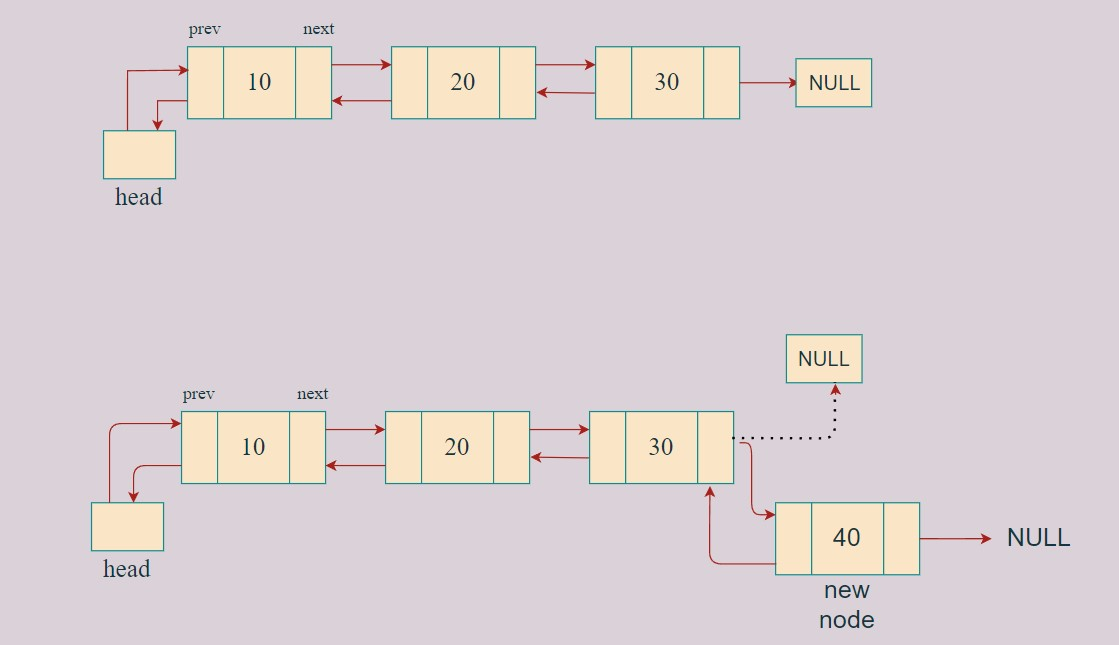# Insert at end of Doubly Linked List

Write program to Insert at end of Doubly Linked List.

Problem Statement: Given a doubly-linked list, insert a node  at the end of the doubly linked list.

Examples:

```Example 1:
Input:  List : 1 <--> 2 <--> 3 <--> 4 <--> 5
K :  6
Output: List :  1 <--> 2 <--> 3 <--> 4 <--> 5 <--> 6
Explanation: the value given in k is inserted at the tail of the doubly linked lists.

Example 2:
Input:  List: NULL
K : 2
Output:	List : 2
Explanation: The list is empty so it will become the first node.```

### Solution

DisclaimerDon’t jump directly to the solution, try it out yourself first.

### Intuition:

To put at the end of the doubly linked list we can point the last node of a doubly linked list to the new node and the previous of the new node to the last node.

### Approach:

To insert at the end of the linked list:

• We will first create a new node.
• We have to traverse to the end of the linked list and then change the next of the last node to a new node.
• If the linked list is empty then make the new node as head of the linked list
• Else traverse to the last node and change the last node then make the last node as previous of new node.Code:

## C++ Code

``````#include<bits/stdc++.h>

using namespace std;

class node {
public:
int data;
node * next;
node * prev;
node(int val) {
data = val;
next = NULL;
prev = NULL;
}
};

void insertAtTail(node * & head, int val) {
node * new_node = new node(val);
if (head == NULL) {
return;
}
node * temp = head;
while (temp -> next != NULL) {
temp = temp -> next;
}
temp -> next = new_node;
new_node -> prev = temp;
}

void display(node * head) {
node * temp = head;
while (temp != NULL) {
cout << temp -> data << "-->";
temp = temp -> next;
}
cout << "NULL\n";
}

int main() {

}
``````

Output: 1–>2–>3–>4–>5–>NULL

Time Complexity: O(n) as, we are inserting in the end so we are traversing  through the list

Space Complexity: O(1)  As, we do not need extra space to store the node.

## Java Code

``````import java.util.*;

class Node {

int data;
Node  next;
Node  prev;
Node(int val) {
data = val;
next = null;
prev = null;
}
}

class TUF{
static Node insertAtTail(Node head, int val) {
Node  new_node = new Node(val);
if (head == null) {
}
Node  temp = head;
while (temp . next != null) {
temp = temp . next;
}
temp . next = new_node;
new_node . prev = temp;
}

static void display(Node  head) {
Node  temp = head;
while (temp != null) {
System.out.print(temp.data+"-->");
temp = temp . next;
}
System.out.println("NULL");
}

public static void main(String args[]) {